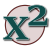Games
Problems
Go Pro!

Lesson Plans > Mathematics > Algebra > Factoring > Quadratic Formula

# Featured Lesson PlansA lesson plan that leads students through a proof of the Quadratic FormulaDerivation of the quadratic formula - how to teach your students why this works

# Full Directory Listing

Proving the Quadratic Formula, Quadratic Formula : Derivation and Application to ProblemsLike us on Facebook to get updates about new resources